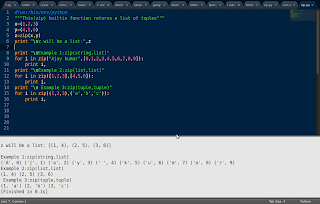## Saturday, March 16, 2013

### ZIP in python with examples

```#!usr/bin/env/python
"""This(zip) builtin function returns a list of tuples"""
x=(1,2,3)
y=(4,5,6)
z=zip(x,y)
print "\nz will be a list:",z

print "\nExample 1:zip(string,list)"
for i in zip("Ajay kumar",[0,1,2,3,4,5,6,7,8,9]):
print i,
print "\nExample 2:zip(list,list)"
for i in zip([1,2,3],[4,5,6]):
print i,
print "\n Example 3:zip(tuple,tuple)"
for i in zip((1,2,3),('a','b','c')):
print i,
```

Output:

```z will be a list: [(1, 4), (2, 5), (3, 6)]

Example 1:zip(string,list)
('A', 0) ('j', 1) ('a', 2) ('y', 3) (' ', 4) ('k', 5) ('u', 6) ('m', 7) ('a', 8) ('r', 9)
Example 2:zip(list,list)
(1, 4) (2, 5) (3, 6)
Example 3:zip(tuple,tuple)
(1, 'a') (2, 'b') (3, 'c')```

Output can be seen in the image` `
` `
`One More version from some online book:`
` `
```z = zip(list1, list2)
newlist1, newlist2 = zip(*z)```

This works becauses the * syntax unpacks a list of values. The above code zips and unzips two lists, which is pointless, but the same syntax can be used to convert from a list of columns of data to a list of rows of data. For example, the following list comprehension reads in a file of tab-delimited data as a list of rows, where each row is a tuple of values:
`rows = [line.rstrip().split('\t') for line in file(filename)]`
If you want to flip the data through 90 degrees (i.e. convert from rows or data to columns of data), then you use:
`columns = zip(*rows)`
For example, if the data was originally (a, 1), (b, 2), (c, 3), it becomes (a, b, c), (1, 2, 3).
Learn python for fun.The popular blog with questions and answers to the python.Solutions to facebookhackercup,codejam,codechef.The fun way to learn python with me.Building some cool apps.